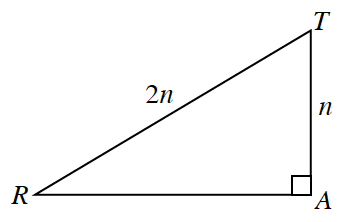### Home > PC > Chapter 3 > Lesson 3.2.2 > Problem3-74

3-74.

Use $\triangle RAT$ below to complete the following problems.1. Find the exact length of $\overline{R A}$ in terms of $n$.

Use the Pythagorean Theorem.

2. Using the triangle, find the ratios for:

1. $\sin R$

2. $\cos R$

3. $\tan R$

SOH CAH TOA

3. Using your results from part (b), find and simplify $\frac { \operatorname { sin } R } { \operatorname { cos } R }$. What do you notice?

${\text{It is the same as }\tan(R) = \frac{1}{\sqrt{3}} = \frac{\sqrt{3}}{3}.}$